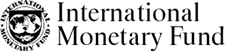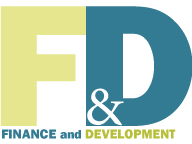﻿ What Is Econometrics? Back to Basics: Finance & Development, December 2011

### What Is Econometrics?

Finance & Development, December 2011, Vol. 48, No. 4

Sam Ouliaris

Taking a theory and quantifying it

ECONOMISTS develop economic models to explain consistently recurring relationships. Their models link one or more economic variables to other economic variables (see “What Are Economic Models,” F&D, June 2011). For example, economists connect the amount individuals spend on consumer goods to disposable income and wealth, and expect consumption to increase as disposable income and wealth increase (that is, the relationship is positive).

There are often competing models capable of explaining the same recurring relationship, called an empirical regularity, but few models provide useful clues to the magnitude of the association. Yet this is what matters most to policymakers. When setting monetary policy, for example, central bankers need to know the likely impact of changes in official interest rates on inflation and the growth rate of the economy. It is in cases like this that economists turn to econometrics.

Econometrics uses economic theory, mathematics, and statistical inference to quantify economic phenomena. In other words, it turns theoretical economic models into useful tools for economic policymaking. The objective of econometrics is to convert qualitative statements (such as “the relationship between two or more variables is positive”) into quantitative statements (such as “consumption expenditure increases by 95 cents for every one dollar increase in disposable income”). Econometricians—practitioners of econometrics—transform models developed by economic theorists into versions that can be estimated. As Stock and Watson (2007) put it, “econometric methods are used in many branches of economics, including finance, labor economics, macroeconomics, microeconomics, and economic policy.” Economic policy decisions are rarely made without econometric analysis to assess their impact.

Certain features of economic data make it challenging for economists to quantify economic models. Unlike researchers in the physical sciences, econometricians are rarely able to conduct controlled experiments in which only one variable is changed and the response of the subject to that change is measured. Instead, econometricians estimate economic relationships using data generated by a complex system of related equations, in which all variables may change at the same time. That raises the question of whether there is even enough information in the data to identify the unknowns in the model.

Econometrics can be divided into theoretical and applied components.

Theoretical econometricians investigate the properties of existing statistical tests and procedures for estimating unknowns in the model. They also seek to develop new statistical procedures that are valid (or robust) despite the peculiarities of economic data—such as their tendency to change simultaneously. Theoretical econometrics relies heavily on mathematics, theoretical statistics, and numerical methods to prove that the new procedures have the ability to draw correct inferences.

Applied econometricians, by contrast, use econometric techniques developed by the theorists to translate qualitative economic statements into quantitative ones. Because applied econometricians are closer to the data, they often run into—and alert their theoretical counterparts to—data attributes that lead to problems with existing estimation techniques. For example, the econometrician might discover that the variance of the data (how much individual values in a series differ from the overall average) is changing over time.

The main tool of econometrics is the linear multiple regression model, which provides a formal approach to estimating how a change in one economic variable, the explanatory variable, affects the variable being explained, the dependent variable—taking into account the impact of all the other determinants of the dependent variable. This qualification is important because a regression seeks to estimate the marginal impact of a particular explanatory variable after taking into account the impact of the other explanatory variables in the model (see “Regressions: Why Are Economists Obsessed with Them?F&D, March 2006). For example, the model may try to isolate the effect of a 1 percentage point increase in taxes on average household consumption expenditure, holding constant other determinants of consumption, such as pretax income, wealth, and interest rates.

#### Stages of development

The methodology of econometrics is fairly straightforward.

The first step is to suggest a theory or hypothesis to explain the data being examined. The explanatory variables in the model are specified, and the sign and/or magnitude of the relationship between each explanatory variable and the dependent variable are clearly stated. At this stage of the analysis, applied econometricians rely heavily on economic theory to formulate the hypothesis. For example, a tenet of international economics is that prices across open borders move together after allowing for nominal exchange rate movements (purchasing power parity). The empirical relationship between domestic prices and foreign prices (adjusted for nominal exchange rate movements) should be positive, and they should move together approximately one for one.

The second step is the specification of a statistical model that captures the essence of the theory the economist is testing. The model proposes a specific mathematical relationship between the dependent variable and the explanatory variables—on which, unfortunately, economic theory is usually silent. By far the most common approach is to assume linearity—meaning that any change in an explanatory variable will always produce the same change in the dependent variable (that is, a straight-line relationship).

Because it is impossible to account for every influence on the dependent variable, a catchall variable is added to the statistical model to complete its specification. The role of the catchall is to represent all the determinants of the dependent variable that cannot be accounted for—because of either the complexity of the data or its absence. Economists usually assume that this “error” term averages to zero and is unpredictable, simply to be consistent with the premise that the statistical model accounts for all the important explanatory variables.

The third step involves using an appropriate statistical procedure and an econometric software package to estimate the unknown parameters (coefficients) of the model using economic data. This is often the easiest part of the analysis thanks to readily available economic data and excellent econometric software. Still, the famous GIGO (garbage in, garbage out) principle of computing also applies to econometrics. Just because something can be computed doesn’t mean it makes economic sense to do so.

The fourth step is by far the most important: administering the smell test. Does the estimated model make economic sense—that is, yield meaningful economic predictions? For example, are the signs of the estimated parameters that connect the dependent variable to the explanatory variables consistent with the predictions of the underlying economic theory? (In the household consumption example, for instance, the validity of the statistical model would be in question if it predicted a decline in consumer spending when income increased). If the estimated parameters do not make sense, how should the econometrician change the statistical model to yield sensible estimates? And does a more sensible estimate imply an economically significant effect? This step, in particular, calls on and tests the applied econometrician’s skill and experience.

#### Testing the hypothesis

The main tool of the fourth stage is hypothesis testing, a formal statistical procedure during which the researcher makes a specific statement about the true value of an economic parameter, and a statistical test determines whether the estimated parameter is consistent with that hypothesis. If it is not, the researcher must either reject the hypothesis or make new specifications in the statistical model and start over.

If all four stages proceed well, the result is a tool that can be used to assess the empirical validity of an abstract economic model. The empirical model may also be used to construct a way to forecast the dependent variable, potentially helping policymakers make decisions about changes in monetary and/or fiscal policy to keep the economy on an even keel.

Students of econometrics are often fascinated by the ability of linear multiple regression to estimate economic relationships. Three fundamentals of econometrics are worth remembering.

• First, the quality of the parameter estimates depends on the validity of the underlying economic model.

• Second, if a relevant explanatory variable is excluded, the most likely outcome is poor parameter estimates.

• Third, even if the econometrician identifies the process that actually generated the data, the parameter estimates have only a slim chance of being equal to the actual parameter values that generated the data. Nevertheless, the estimates will be used because, statistically speaking, they will become precise as more data become available.

Econometrics, by design, can yield correct predictions on average, but only with the help of sound economics to guide the specification of the empirical model. Even though it is a science, with well-established rules and procedures for fitting models to economic data, in practice econometrics is an art that requires considerable judgment to obtain estimates useful for policymaking. ■

Sam Ouliaris is a Senior Economist in the IMF Institute.

#### Reference

Stock, James H., and Mark W. Watson, 2007, Introduction to Econometrics, Addison-Wesley Series in Economics (Boston: Pearson Addison Wesley, 2nd ed.).#### Write to us

F&D welcomes comments and brief letters, a selection of which are posted under Letters to the Editor. Letters may be edited. Please send your letters to fanddletters@imf.org